Skip to main content

# A blackbox yield estimation workflow with Gaussian process regression applied to the design of electromagnetic devices

## Abstract

In this paper an efficient and reliable method for stochastic yield estimation is presented. Since one main challenge of uncertainty quantification is the computational feasibility, we propose a hybrid approach where most of the Monte Carlo sample points are evaluated with a surrogate model, and only a few sample points are reevaluated with the original high fidelity model. Gaussian process regression is a non-intrusive method which is used to build the surrogate model. Without many prerequisites, this gives us not only an approximation of the function value, but also an error indicator that we can use to decide whether a sample point should be reevaluated or not. For two benchmark problems, a dielectrical waveguide and a lowpass filter, the proposed methods outperform classic approaches.

## Introduction

In mass production of electrical devices, e.g. antennas or filters, often one has to deal with uncertainties in the manufacturing process. These uncertainties may lead to deviations in important parameters, e.g. geometrical or material parameters. Those may lead to rejections due to malfunctioning. In this context, the quantification of uncertainty and its impact plays an important role, also with regard to later optimization. According to Graeb [1, Chap. 2] we define the yield as the percentage of realizations in a manufacturing process, which fulfills performance feature specifications. When dealing with electromagnetism, the performance feature specifications are requirements involving partial differential equations (PDEs) describing the electromagnetic fields, i.e., Maxwell’s equations. These can be solved numerically, e.g. with the finite element method (FEM). The most straightforward approach for yield estimation is the Monte Carlo (MC) analysis [2, Chap. 5]. The space of the uncertain parameters is sampled and the performance feature specifications are tested for each sample point. This requires typically many evaluations of the underlying PDEs. Thus, the computational effort is one main challenge of yield estimation.

Over the last decades, various methods have been developed with the aim of reducing the computational effort of MC. One approach to achieve this is to reduce the number of sample points, e.g. by Importance Sampling (IS) [2, Chap. 5.4]. Another approach is to reduce the effort for each sample point, e.g. by using surrogate based approaches . The cost for building most surrogate models increases rapidly with the number of uncertain parameters. Furthermore, there are counter examples where the yield estimator fails drastically, even though the surrogate model seems highly accurate, measured by classical norms or pointwise . Therefore the same authors propose a hybrid approach. They focus their attention on critical sample points that are close to the limit state function, which is the limit between sample points fulfilling and not fulfilling the performance feature specifications. Critical sample points are evaluated on the original high fidelity model, while the other sample points that are far from the limit state function are evaluated only on a surrogate model. Because of this distinction, which leads to a combination of different model types, it is called a hybrid approach. Here, the choice of the surrogate model and the definition of close and far are crucial. In , a hybrid approach is proposed, using radial basis functions (RBF) for the surrogate model and an adjoint error indicator to choose the critical sample points. In  a similar hybrid approach is proposed, using stochastic collocation with polynomial basis functions and also an adjoint error indicator. In this paper we combine these ideas and propose a hybrid approach using Gaussian process regression (GPR) for both, building the surrogate model and obtaining an error indicator in form of the prediction standard deviation given by the GPR. The critical sample points are used to improve the GPR model adaptively during the estimation process. Further we investigate if sorting the sample points can increase the efficiency.

Other research related to GPR based surrogate models for yield or failure probability estimation is conducted in . In  various sorting strategies for GPR model training data are described and compared. In , the authors concentrate on the calculation of small failure probabilities with a limited number of function evaluations on the high fidelity model. They also use an adaptive GPR surrogate model, but do not combine it with a hybrid approach and therefore have no critical sample points that could be used to improve the GPR model. Instead, they distinguish between the sample points generated by Subset Simulation (Sequential MC) for error probability estimation and those generated as training data using a Stepwise Uncertainty Reduction technique to refine the GPR model adaptively. In  and , a GPR based surrogate model approach is combined with IS. Again, no hybrid approach is used. Adaptively, GPR model and IS density are improved by adding one or more sample points from the MC sample of the last iteration to the training data set, which are selected by a learning function and then calculated on the high fidelity model. On the contrary, in practice it is often assumed that the design parameter deviations are small in a way that a linearization is valid [13, Online Help: Yield Analysis Overview]. This approach is obviously very efficient but it is very difficult to determine on beforehand if the assumption is valid.

The paper is structured as follows. After setting up the problem, in Sect. 3 existing approaches and the concept of GPR are briefly described. Then the use of GPR for yield estimation, also in combination with a hybrid approach, is discussed. In Sect. 4, numerical results are presented using a benchmark problem, a simple waveguide, and a practical example, a low pass filter calculated with CST, before the paper is concluded in Sect. 5.

## Problem setting

Even though the proposed ideas are generally applicable, in the following we will focus on problems from the electrical engineering where electromagnetic field simulations are necessary. This is the case, for example, when designing antennas or filters. Depending on the frequency, e.g. the electric field can be calculated to retrieve information about the performance of the device. Then the performance can be optimized by adapting the design. In order to calculate the electric field on a simply connected bounded domain D, we start from Maxwell’s formulation

$$\nabla \times \bigl(\mu ^{-1} \nabla \times \mathbf {E}_{\omega } \bigr) - \omega ^{2} \varepsilon \mathbf {E}_{\omega } = j \omega \mathbf {J}\quad \text{on }D,$$

where $$\mathbf {E}_{\omega }= \mathbf {E}_{\omega }(\mathbf {x}, \mathbf {p})$$ denotes the electric field phasor, ω the angular frequency, $$\mu =\mu _{r} \mu _{0}$$ the dispersive complex magnetic permeability, $$\varepsilon =\varepsilon _{r}\varepsilon _{0}$$ the dispersive complex electric permittivity and $$\mathbf {J}=\mathbf {J}(\mathbf {x},\mathbf {p})$$ the phasor of current density. The vacuum and relative permeability are denoted by $$\mu _{0}$$ and $$\mu _{r}=\mu _{r}(\mathbf {x},\mathbf {p})$$, the vacuum and relative permittivity respectively by $$\varepsilon _{0}$$ and $$\varepsilon _{r}=\varepsilon _{r}(\mathbf {x},\mathbf {p})$$. Assuming suitable boundary conditions, building the weak formulation and discretizing with (high-order) Nédélec basis functions we derive the linear system

$$\mathbf {A}_{\omega } (\mathbf {p}) \mathbf {e}_{\omega }(\mathbf {p}) = \mathbf {f}_{\omega },$$
(1)

with system matrix $$\mathbf {A}_{\omega } (\mathbf {p})$$, discrete solution $$\mathbf {e}_{\omega }(\mathbf {p})$$, the discretized right-hand side $$\mathbf {f}_{\omega }$$, all depending on the design parameter p and the frequency ω. For further details we refer to . As quantity of interest (QoI)

$$Q_{\omega }(\mathbf {p}) = q \bigl( \mathbf {e}_{\omega }(\mathbf {p}) \bigr),$$

we consider the scattering parameter (S-parameter), cf. [17, Chap. 3] and , i.e., $$Q_{\omega }(\mathbf {p}) := S_{\omega }(\mathbf {p})$$. In this case, q is an affine linear function, but this is no requirement for the following yield estimation methods.

If there are uncertainties in the manufacturing process, the design parameters may be subject to random deviations. Therefore we model the uncertain parameter vector p as multidimensional random variable. We assume p to be (truncated) Gaussian distributed (cf. ), i.e., $$\mathbf {p}\sim {\mathcal{N_{T}} ( \overline{\mathbf {p}}, \boldsymbol {\Sigma}, \mathbf {lb} , \mathbf {ub} )}$$ with mean value $$\overline{\mathbf {p}}$$, covariance matrix Σ, lower and upper bounds lb and ub and the corresponding probability density function 

$$\operatorname {pdf}_{\mathcal{N_{T}}}(\mathbf {p}) = \textstyle\begin{cases} \frac{\mathrm{e}^{-\frac{1}{2}(\mathbf {p}-\overline{\mathbf {p}})^{\top } \boldsymbol {\Sigma }^{-1} (\mathbf {p}-\overline{\mathbf {p}})} }{\int _{\mathbf {lb}}^{\mathbf {ub}} \mathrm{e}^{-\frac{1}{2}(\mathbf {p}-\overline{\mathbf {p}})^{\top } \boldsymbol {\Sigma }^{-1} (\mathbf {p}-\overline{\mathbf {p}})} \,\mathrm {d}\mathbf {p}} & \text{if } \mathbf {lb} \leq \mathbf {p}\leq \mathbf {ub}, \\ 0 & \text{else}. \end{cases}$$

Note that the definition of the yield and the proposed estimation method is independent of the chosen probability density function $$\operatorname {pdf}(\mathbf {p})$$. The Gaussian distribution is a typical choice for modeling design parameters as uncertain. Here, we truncate it to avoid non-physical realizations of p, e.g. negative distances. Following  we define the performance feature specifications as a restriction on our QoI in a specific interval, i.e.,

$$\bigl\vert S_{\omega }(\mathbf {p}) \bigr\vert \leq c\quad \forall \omega \in T_{\omega } = [\omega _{\mathrm{l}},\omega _{\mathrm{u}} ] \text{ in GHz},$$
(2)

where c is a constant and ω a range parameter from an interval $$T_{\omega }$$. Here, we identify ω with the frequency and $$T_{\omega }$$ with the frequency domain of interest. The safe domain is defined as the set containing all parameters, fulfilling the performance feature specifications, i.e.,

$$\Omega _{\mathrm {s}}:= \bigl\lbrace \mathbf {p}: \bigl\vert S_{\omega }(\mathbf {p}) \bigr\vert \leq c\ \forall \omega \in T_{\omega } \bigr\rbrace .$$
(3)

Then, the yield can be written as [1, Chap. 4.8.3, Eq. (137)]

$$Y(\overline{\mathbf {p}}) := \mathbb {E}\bigl[\mathbf {1}_{\Omega _{\mathrm {s}}}(\mathbf {p})\bigr] := \int _{- \infty }^{\infty } \cdots \int _{-\infty }^{\infty } \mathbf {1}_{\Omega _{\mathrm {s}}}( \mathbf {p}) \operatorname {pdf}(\mathbf {p}) \,\mathrm {d}\mathbf {p},$$

where $$\mathbb {E}$$ denotes the expected value and $$\mathbf {1}_{\Omega _{\mathrm {s}}}$$ the indicator function with value 1 if the parameter p lies inside the safe domain and value 0 otherwise.

## A GPR-hybrid approach for yield estimation

In a MC analysis a large number of sample points is generated, according to the truncated normal distribution of the uncertain parameters, and evaluated in order to obtain the QoI. The fraction of sample points lying inside the safe domain is an estimator for the yield. Since the accuracy depends directly on the size of the sample, a classic MC analysis comes with high computational costs . In the past, various surrogate based approaches have been proposed. The idea is to approximate the QoI, i.e., find a mapping from the design parameter p to $$\tilde{S}_{\omega }$$, where $$\tilde{S}_{\omega }$$ is an approximation of $$S_{\omega }$$. This allows to evaluate the performance feature specifications (2) and thus the safe domain (3) without solving a PDE for each sample point. The stochastic collocation hybrid approach proposed by  showed that the computational effort can be reduced significantly while ensuring the same accuracy and robustness as with a classic MC method. Nevertheless, there are a few drawbacks. First, since a polynomial collocation approach was used, the training data for the surrogate model must come from a tensorial grid and cannot be chosen arbitrarily. As a consequence the surrogate model cannot be updated easily, e.g. with the information from the evaluation of critical sample points. This could be handled by using regression, but the second disadvantage would still remain: In order to distinguish between critical and non-critical sample points an adjoint error indicator was used. This requires the system matrices and the solution of the primal and the dual problem, which is not always given when using proprietary software and can become very costly in case of non-linear QoIs. The GPR-Hybrid approach we propose in this paper overcomes these issues.

### Gaussian process regression

Following Rasmussen and Williams [4, Chap. 2.2], the technique of Gaussian process regression can be divided into four mandatory steps and one optional step.

### 1. Prior:

We make some prior assumptions about the functions we expect to observe. We write

$$(S_{\mathbf {p}})_{\mathbf {p}\in \mathbf {P}} \sim \mathcal{GP} \bigl( m( \mathbf {p}),k \bigl(\mathbf {p},\mathbf {p}' \bigr) \bigr),$$

if we expect the S-parameter to follow a Gaussian process (GP) with specific mean m and kernel function k. In the following we use the constant zero function as a starting value for the mean function. When the GP is trained, the mean value of the training data evaluations will be used. As kernel function we choose the squared exponential kernel, which is also known as RBF, i.e.,

$$k \bigl(\mathbf {p},\mathbf {p}' \bigr) = \zeta \mathrm{e}^{- \frac{ \vert \mathbf {p}-\mathbf {p}' \vert ^{2}}{2l^{2}}},$$

with the two hyperparameters $$\zeta \in \mathbb {R}$$ and $$l>0$$. At this point we refer to Sect. 4 to see how we set the hyperparameters. For more information about hyperparameters in general, please refer to [4, Chap. 5].

### 2. Training data:

We collect data by evaluating sample points on the high fidelity FE model. The so-called training data set

$$\mathcal{T}= \bigl\lbrace \mathbf {P}=[\mathbf {p}_{1},\dots ,\mathbf {p}_{n}]^{{ \top }}, \mathbf {S} = \bigl[S(\mathbf {p}_{1}),\dots ,S( \mathbf {p}_{n})\bigr]^{{\top }} \bigr\rbrace$$

will be used to train the GP. In the following, we generate these sample points according to the distribution of the uncertain parameters.

### 3. Posterior:

In this step the information from the prior and the training data is combined in order to obtain a new GP, with updated mean and kernel function. We write

$$\mathbf {K} = \begin{bmatrix} k(\mathbf {p}_{1},\mathbf {p}_{1}) & \cdots & k(\mathbf {p}_{1},\mathbf {p}_{n}) \\ \vdots & & \vdots \\ k(\mathbf {p}_{n},\mathbf {p}_{1}) & \cdots & k(\mathbf {p}_{n},\mathbf {p}_{n}) \end{bmatrix} \quad \text{and}\quad \mathbf {m} = \begin{bmatrix} m(\mathbf {p}_{1}) \\ \vdots \\ m(\mathbf {p}_{n}) \end{bmatrix},$$
(4)

then the posterior distribution of the output $$S_{\mathbf {p}}$$ depending on the training data set $$\mathcal{T}$$ is given by

$$S_{\mathbf {p}}|\mathbf {P}, \mathbf {S} \sim \mathcal{N}(\mathbf {m}, \mathbf {K}).$$

### 4. Predictions:

For an arbitrary test data point $$\mathbf {p}^{\star }$$ the predicted distribution of the output $$S_{\mathbf {p}^{\star }}$$ depending on the training data set $$\mathcal{T}$$ and the test data point is given by

\begin{aligned} S_{\mathbf {p}^{\star }}|\mathbf {p}^{\star }, \mathbf {P}, \mathbf {S} &\sim \mathcal{N} \bigl( m\bigl(\mathbf {p}^{\star }\bigr) + \mathbf {k}\bigl( \mathbf {p}^{\star }, \mathbf {P}\bigr) \mathbf {K}^{-1} (\mathbf {S} - \mathbf {m}), \\ &\quad k\bigl(\mathbf {p}^{\star },\mathbf {p}^{\star }\bigr)-\mathbf {k} \bigl(\mathbf {p}^{\star }, \mathbf {P}\bigr)\mathbf {K}^{-1} \mathbf {k}\bigl(\mathbf {P},\mathbf {p}^{\star }\bigr) \bigr), \end{aligned}
(5)

with

\begin{aligned}& \mathbf {k}\bigl(\mathbf {p}^{\star },\mathbf {P}\bigr) = \bigl[k\bigl(\mathbf {p}^{\star },\mathbf {p}_{1}\bigr),\dots ,k \bigl(\mathbf {p}^{\star }, \mathbf {p}_{n}\bigr) \bigr], \\& \mathbf {k}\bigl(\mathbf {P},\mathbf {p}^{\star }\bigr) = \bigl[k\bigl(\mathbf {p}_{1},\mathbf {p}^{\star }\bigr),\dots ,k \bigl(\mathbf {p}_{n}, \mathbf {p}^{\star }\bigr) \bigr]^{\top }. \end{aligned}

Thus, GPR predictions of the function value $$\tilde{S}_{\text{GPR}}(\mathbf {p}^{\star })$$ and the standard deviation $$\sigma _{\text{GPR}}(\mathbf {p}^{\star })$$ can be obtained. Please note, that $$\sigma _{\text{GPR}}(\mathbf {p}^{\star })$$ is the standard deviation of the surrogate model and is not related to the design uncertainty, i.e., Σ.

### 5. Model update (optional):

A new data point $$(\mathbf {p}_{\text{add.}}, S(\mathbf {p}_{\text{add.}}))$$ can be used to update an existing GPR model. Therefore the training data set is updated to

$$\mathbf {P}_{\text{new}} = [\mathbf {P}, \mathbf {p}_{\text{add.}}] \quad \text{and}\quad \mathbf {S}_{\text{new}} = \bigl[\mathbf {S}, S(\mathbf {p}_{\text{add.}})\bigr],$$
(6)

as well as (4) has to be updated according to

$$\mathbf {K}_{\text{new}} = \begin{bmatrix} \mathbf {K} & \mathbf {k}(\mathbf {P},\mathbf {p}_{\text{add.}}) \\ \mathbf {k}(\mathbf {p}_{\text{add.}}, \mathbf {P}) & k(\mathbf {p}_{\text{add.}}, \mathbf {p}_{\text{add.}}) \end{bmatrix}\quad \text{and} \quad \mathbf {m}_{\text{new}} = \begin{bmatrix} \mathbf {m} \\ m(\mathbf {p}_{\text{add.}}) \end{bmatrix}.$$
(7)

Then, predictions for a new test data point $$\mathbf {p}^{\star }$$ can be obtained by (5) using the updated data from (6) and (7). This update involves the factorization of the matrix $$\mathbf {K}_{\text{new}}$$ which is in the worst case of cubic complexity, i.e., $$\mathcal{O}(n^{3})$$, and can be reduced to $$\mathcal{O}(r^{2}n)$$ by using low-rank approximations, where n is the number of training data points and r the rank of the low-rank approximation [4, Chap. 8]. In conclusion, we assume that this effort is negligible in comparison to the high fidelity evaluations, i.e., solving (1). For more detailed information about GPR we refer to [4, Chap. 2].

### Combining GPR and the hybrid approach

The idea of the hybrid approach is saving computing time by evaluating most of the MC sample points on a cheap to evaluate surrogate model and only a small subset of the sample on the original high fidelity (e.g. FE) model. The critical sample points, i.e., sample points close to the limit state function, are those which are evaluated on the high fidelity model. As mentioned before, the choice of the critical sample points is crucial, for efficiency and accuracy of this approach. In  and  adjoint error indicators are used. Here, we take advantage of the GPR that provides an error indicator in the point p in the form of the standard deviation $$\sigma _{\text{GPR}}(\mathbf {p})$$. The performance feature specification expects the inequality (2) to hold in the whole frequency interval $$T_{\omega }$$. However, we define a discrete subset $$T_{\text{d}} \subset T_{\omega }$$ and enforce only that the inequality holds for all $$\omega _{j} \in T_{\text{d}}$$. This means, for each frequency point $$\omega _{j}$$ a separate surrogate model is built, otherwise rational interpolation could be used, e.g. . Thus, the GPR model and the resulting prediction values and standard deviations depend on the frequency and are denoted by $$\tilde{S}_{\text{GPR},\omega _{j}}(\mathbf {p})$$ and $$\sigma _{\text{GPR},\omega _{j}}(\mathbf {p})$$ with $$j=1,\dots ,|T_{\text{d}}|$$. We apply a short circuit strategy, i.e., a sample point is not evaluated on the remaining frequency points if it has already been rejected for a previous one. This allows us to save computing time and does not affect the estimation result, except the case that a sample point has been rejected erroneously based on an underestimated standard deviation prediction. Further, we build separate surrogate models for the real part and the imaginary part of the S-parameter, and later combine them for the prediction. This guarantees (affin-)linearity of the QoI by avoiding the square root. Algorithm 1 shows the classification procedure for one sample point $$\mathbf {p}_{i}$$. Once the GPR models are constructed, a MC analysis is carried out on the surrogates. For each sample point a predicted S-parameter value and a predicted standard deviation are obtained. Following the concept of sigma-levels , the predicted standard deviation multiplied with a safety factor γ is considered as an error indicator for the surrogate model. The value of γ is problem dependent and can be derived by evaluating some test data points on the high fidelity model and on the GPR model and considering the ratio of the true error and the predicted error, i.e., the standard deviation. The predicted standard deviation multiplied with the safety factor γ serves as a buffer zone. If the performance feature specification (2) is (not) fulfilled for the predicted S-parameter value and all values in the range plus/minus this buffer zone the considered sample point is classified as (not) accepted, else it is classified as critical and reevaluated on the high fidelity model. Then, the yield will be estimated by

$$\tilde{Y}(\overline{\mathbf {p}}) = \frac{1}{N_{\mathrm {MC}}} \sum _{i=1}^{N_{\mathrm {MC}}} \mathbf {1}_{\Omega _{\mathrm {s}}}(\mathbf {p}_{i}),$$

where $$N_{\mathrm {MC}}$$ is the size of the MC sample. A significant advantage of GPR is, that the model can be easily updated on the fly. Algorithm 2 shows the process of yield estimation including updating the GPR models. Typically the computational effort of a surrogate based approach lies in the offline evaluation of the training data. Therefore we start with a small initial training data set. The resulting less accurate GPR model does not pose a problem in terms of yield estimation accuracy, because the hybrid method still classifies all MC sample points correctly as accepted or not accepted. The only difference is, that there might be more critical sample points in the beginning, if the initial GPR surrogate has been built with a smaller training data set. Then, during the estimation process (online), we use critical sample points to improve our GPR model. This update requires almost no additional computational effort, since these sample points were calculated in the hybrid method anyway. In order to enable parallel computing even with model updates, we introduce so-called batches. Only after the calculation of $$N_{\text{B}}$$ high fidelity evaluations (possibly in parallel), a GPR model update is considered. With $$N_{\text{B}}$$ we refer to the size of the batches, setting $$N_{\text{B}}=1$$ indicates that no batches are used. If only a part of the critical sample points is added to the training data set for updating the GPR model, they are chosen in a greedy way: After evaluating one batch of MC sample points, the resulting critical sample points of the j-th frequency point are collected in the set $$\mathcal{C}_{j}$$ (cf. line 6 in Algorithm 2). Then, the sample point for which the difference between the predicted value and the real value of the S-parameter is maximum will be included in the training data set (cf. line 12 in Algorithm 2). The GPR model is updated with the additional training data point and all sample points in $$\mathcal{C}_{j}$$ are evaluated on the new GPR surrogate model in order to obtain a new prediction. This procedure is repeated until the error is below a tolerance $$\varepsilon _{t}$$. Using the updated GPR model, the next MC sample points are evaluated until again $$N_{\text{B}}$$ sample points have been evaluated on the high fidelity model (in parallel) and GPR model updates are considered. Without much extra cost it is also possible to reevaluate all already considered, non-critical sample points after each GPR model update. At this point it can also be decided whether all critical sample points are added to the training data set or only a part. Especially when solving in batches, it can be advisable not to include all critical sample points in order to avoid adding to many, closely neighboring sample points. If $$\varepsilon _{t}=0$$, all critical sample points are used to update the GPR model.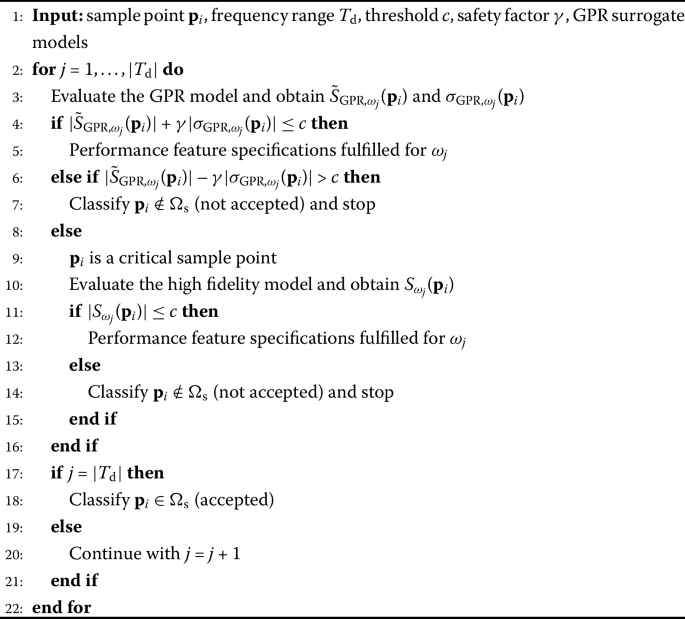Algorithm 1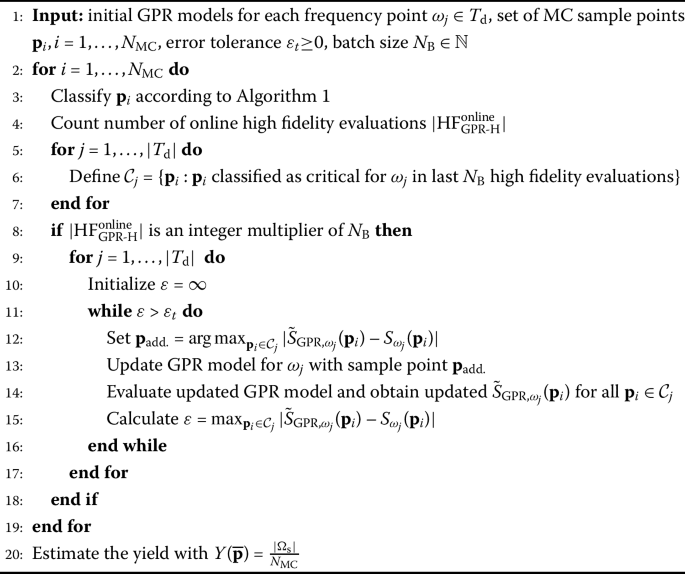Algorithm 2

The proposed updating strategy can be modified by sorting the sample points with negligible costs. The idea is to start with the most promising sample points, i.e., those that contribute most to the improvement of the GPR model. This shall lead to more sample points classified correctly without being evaluated on the high fidelity model. In  different sorting criterions are described and compared. Here, we will focus on two criterions. The criterion proposed by Echard, Gayton and Lemaire in , which we will call the EGL criterion in the following, and a criterion based on our hybrid decision rule, which we will call the Hybrid criterion. The EGL criterion is given by

$$C_{\text{EGL}}(\mathbf {p}_{i}) := \min_{\omega _{j}} \frac{ \vert \tilde{S}_{\text{GPR},\omega _{j}}(\mathbf {p}_{i}) - c \vert }{ \vert \sigma _{\text{GPR},\omega _{j}}(\mathbf {p}_{i}) \vert },$$

where c denotes the upper bound for the performance feature specification from (2). Then, the sample points are sorted such that we start with the smallest value, i.e., $$\min_{\mathbf {p}_{i}} C_{\text{EGL}}(\mathbf {p}_{i})$$ . The Hybrid criterion is defined by

\begin{aligned} C_{\text{H}}(\mathbf {p}_{i}) :={}& \max_{\omega _{j}} \Bigl( c-\bigl( \bigl\vert \tilde{S}_{\text{GPR},\omega _{j}}(\mathbf {p}_{i}) \bigr\vert - \gamma \bigl\vert \sigma _{ \text{GPR},\omega _{j}}( \mathbf {p}_{i}) \bigr\vert \bigr) \bigr) \\ & \bigl( \bigl( \bigl\vert \tilde{S}_{\text{GPR},\omega _{j}}(\mathbf {p}_{i}) \bigr\vert + \gamma \bigl\vert \sigma _{\text{GPR},\omega _{j}}( \mathbf {p}_{i}) \bigr\vert \bigr) -c \Bigr). \end{aligned}

Per definition

$$C_{\text{H}}(\mathbf {p}_{i}) \textstyle\begin{cases} > 0, &\text{if } \mathbf {p}_{i} \text{ is critical}, \\ \leq 0, & \text{else} \end{cases}$$

holds. Using this criterion, the sample points are sorted such that we start with the largest value, i.e., $$\max_{\mathbf {p}_{i}} C_{\text{H}}(\mathbf {p}_{i})$$. Algorithm 3 is a modification of Algorithm 2 including the sorting strategy. Before the classification of each sample point is started, all sample points are evaluated on the GPR models and sorted according to the chosen sorting criterion, e.g. the EGL criterion or the Hybrid criterion. Nevertheless, in the sampling strategy proposed in Algorithm 3 the sorting criterion can be replaced by any other criterion. For the use of batches, for example, a sorting criterion avoiding closely lying sample points within a batch could be even more efficient. After updating the GPR model for one batch of MC sample points, the remaining MC sample points are reevaluated on the updated GPR model and sorted again, according to the chosen criterion. This is repeated until all sample points are classified.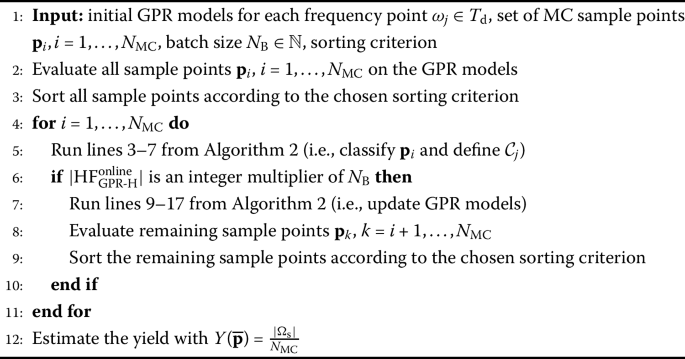Algorithm 3

## Numerical results

In the following we perform numerical tests on two examples, a dielectrical waveguide and a stripline low pass filter. The results of the waveguide are also compared with the estimates resulting from a linearization, which is common in industry. The computations have been carried out with the following configuration: Intel i7-8550U processor with four cores, 1.80 GHz and 16 GB RAM. For solving the corresponding PDEs (1) with FEM, the frequency domain solver of CST Studio Suite®2018  has been used. The yield estimation has been carried out in python 3.7, using the scikit-learn package version 0.21.3  for GPR. Solving our simple models takes only about 15 seconds in CST, while the factorization for the GPR model update is always 1 second.

### Dielectrical waveguide

The benchmark problem, an academic example, on which we perform the numerical tests is a simple dielectrical waveguide, cf. . We consider two uncertain geometrical parameters, the length of the dielectrical inlay $$p_{1}$$, the length of the offset $$p_{2}$$ (see Fig. 1), and two uncertain material parameters $$p_{3}$$ and $$p_{4}$$ with the following effect on the relative permeability and permittivity of the inlay

\begin{aligned}& \varepsilon _{r} = 1+p_{3} + (1-p_{3} ) \bigl(1+j\omega \bigl( 2 \pi 5 \cdot 10^{9} \bigr)^{-1} \bigr)^{-1}, \\& \mu _{r} = 1 + p_{4} + (2-p_{4} ) \bigl(1+j\omega \bigl( 1.1 \cdot 2\pi 20 \cdot 10^{9} \bigr)^{-1} \bigr)^{-1}. \end{aligned}

The mean and covariance (in mm) is given by

$$\overline{\mathbf {p}} = [10.36, 4.76, 0.58, 0.64]^{\top } \quad \text{and}\quad \boldsymbol {\Sigma} = \operatorname{diag} \bigl(\bigl[0.7^{2}, 0.7^{2}, 0.3^{2}, 0.3^{2}\bigr] \bigr).$$

The distribution of the geometrical parameters is truncated on the left at $$p_{i}-3\text{ mm}$$ and on the right at $$p_{i}+3\text{ mm}$$ ($$i=1,2$$), the distribution of the material parameters is truncated on the left at $$p_{i}-0.3$$ and on the right at $$p_{i}+0.3$$ ($$i=3,4$$). The performance feature specifications are

$$\bigl\vert S_{\omega }(\mathbf {p}) \bigr\vert \stackrel{!}{\leq } -24 \text{ dB}\quad \forall \omega \in T_{\omega } = [ 2 \pi 6.5, 2 \pi 7.5 ] \text{ in GHz}.$$

In this frequency range we consider eleven equidistant frequency points $$\omega _{j} \in T_{\text{d}}$$. A commonly used error indicator for MC estimation is given by 

$$\tilde{\sigma }_{Y} := \sqrt{ \frac{Y(\overline{\mathbf {p}})(1-Y(\overline{\mathbf {p}}))}{N_{\mathrm {MC}}}} \leq \frac{0.5}{\sqrt{N_{\mathrm {MC}}}},$$
(8)

where $$\tilde{\sigma }_{Y}$$ denotes the standard deviation of the yield estimator. Since the size of the yield is not known on beforehand, we estimate its upper bound by $$Y(\overline{\mathbf {p}})=0.5$$. We allow a standard deviation of $$\tilde{\sigma }_{Y}=0.01$$. According to (8) this leads to a sample size of $$N_{\mathrm {MC}}= 2500$$. Figure 2 shows values of MC yield estimators of the waveguide for different sample sizes. The black line indicates the most accurate solution we have calculated, i.e., $$Y_{\text{MC}}$$ for $$N_{\mathrm {MC}}=10\text{,}000$$. The gray shaded area indicates the $$\tilde{\sigma }_{Y}$$ level for the yield estimator of the corresponding sample size.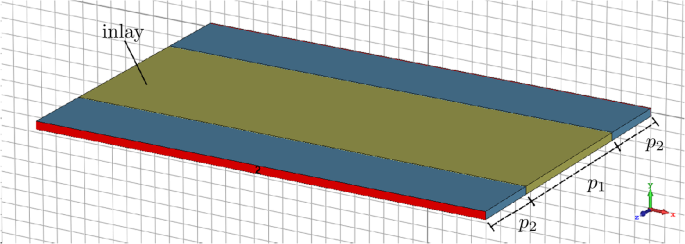Figure 1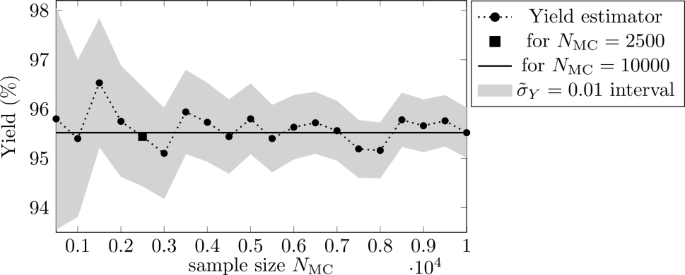Figure 2

The number of high fidelity evaluations before a possible update of the GPR model (batch) can be set to the number of parallel processors available, since these evaluations can be carried out in parallel. However, this value also has another effect: a small number leads to more frequent model updates than a larger number. In general, more frequent model updates imply less critical sample points. We present tests with $$N_{\text{B}}=50$$, $$N_{\text{B}}=20$$ and $$N_{\text{B}}=1$$. The latter implies that no calculation in batches is used. The error tolerance is set to $$\varepsilon _{t}=0$$, since this leads to the best results for the waveguide example, i.e., all critical sample points are added to the training data set. Further we set the safety factor $$\gamma =2$$. This is a rather conservative choice, which may result in too many sample points classified as critical and evaluated on the high fidelity model. Thus, this may increase the computing effort, but it also leads to higher accuracy, since misclassification of sample points is avoided.

For the GPR, the applied kernel is the product of a constant kernel representing ζ and an RBF kernel representing the exponential function with hyperparameter l. In scikit-learn, the hyperparameters have a starting point, in our case $$\zeta _{0} = 0.1$$ and $$l_{0} = 1$$, and then they are optimized within given bounds, in our case $$b_{\zeta } = (10^{-5}, 10^{-1})$$ and $$b_{l}=(10^{-5}, 10^{5})$$, respectively. We allowed the hyperparameters to be tuned within 10 iteration steps in order to find the most suitable values for our data. Due to this optimization, the initial setting does not affect the results of the yield estimation significantly. Further we set the noise parameter $$\alpha = 10^{-5}$$. This parameter defines the allowed deviation from the training data in the interpolation and is recommended to avoid numerical issues, e.g. due to mesh noise. For more information about setting the hyperparameters we refer to [4, Chap. 2.3] and . Once we have evaluated first training data points, the training data’s mean is set as mean function of the GP.

For the simple waveguide a closed form solution of (1) exists, cf. . However, we will refer to this solution as high fidelity solution in the following, since in practice a computational expensive FEM evaluation would be necessary at this point. In the following we denote the number of high fidelity evaluations for a specific method with $$|\text{HF}_{\text{method}}|$$. Further we introduce the number of effective evaluations $$|\text{EE}_{\text{method}}| = \lceil \frac{|\text{HF}_{\text{method}}|}{N_{\text{B}}} \rceil$$, which refers to the non-parallel high fidelity evaluations when using batches. The yield estimator with a pure, classic MC method serves as reference solution $$\tilde{Y}_{\text{Ref.}} = 95.44\%$$. The number of high fidelity evaluations is $$|\text{HF}_{\text{Ref.}}| = 26,360$$. Allowing parallel computing the number of effective evaluations would be 528 for batch size $$N_{\text{B}}=50$$ and 1318 for batch size $$N_{\text{B}}=20$$. Please note, that the short circuit strategy mentioned in Sect. 3.2 has been applied, i.e., a sample point is not tested for a frequency point if it has been rejected for a previous one. Without this short circuit strategy the number of high fidelity evaluations would be the product of the number of frequency points and the size of the MC sample, i.e., $$|T_{\text{d}}| \cdot N_{\mathrm {MC}}= 11\cdot 2500=27\text{,}500$$.

In order to build the GPR models, an initial training data set is needed. It consists of random data points generated according the truncated Gaussian distribution $${\mathcal{N_{T}} ( \overline{\mathbf {p}}, \boldsymbol {\Sigma }, \mathbf {lb} , \mathbf {ub} )}$$ of the uncertain parameters. The size of the initial training data set is chosen, such that the total costs, i.e., the sum of offline (initial training data) and online (critical sample points) costs, is minimal. Using batch size $$N_{\text{B}}=50$$, we tested different sizes of the initial training data set, see Table 1. We proceed with the best performing number of ten training data points. For smaller initial training data sets the offline costs decrease, but the online costs increase. For larger initial training data sets it is the opposite. Only this initial training data set is the same for all GPR models. Then, the estimation procedure with Algorithm 2 is started. After a batch of $$N_{\text{B}}$$ critical sample points the GPR models are updated individually if there were critical sample points on them. Table 2 shows the online high fidelity costs $$|\text{HF}_{\text{GPR-H}}^{\text{online}}|$$ and effective evaluations $$|\text{EE}_{\text{GPR-H}}^{\text{online}}|$$ for yield estimation with different updating strategies. In order to obtain the total costs, the costs for the initial training data set $$|\text{HF}_{\text{GPR-H}}^{\text{offline}}|=110$$, $$|\text{EE}_{\text{GPR-H}}^{\text{offline}}|= \lceil \frac{110}{N_{\text{B}}} \rceil$$ respectively, need to be added. In all cases, the yield estimator is $$\tilde{Y}_{\text{GPR-H}} = 95.44\%$$, so we obtain the same accuracy as with pure MC. With all updating strategies, the number of high fidelity evaluations can be reduced at least by factor 78, in the best case by factor 111, compared to classic MC. In the first setting, $$N_{\text{B}}=1$$, there are no batches (i.e., batches of size 1). Without sorting and with both sorting criteria, this setting has the lowest number of high fidelity evaluations. However, parallel computing is not possible without batches, so the number of effective evaluations equals the number of high fidelity evaluations. Using batches, the GPR models are not updated immediately, only after evaluating the complete batch. This leads to an increasement of the number of high fidelity evaluations. But, batches allow parallel computing (on $$N_{\text{B}}$$ parallel computers), i.e., the number of effective evaluations is much lower.

Further, we see that the number of high fidelity evaluations decreases when applying a sorting strategy. The GPR model is improved after the evaluation of a critical sample point. Due to the sorting, we start with the most critical sample points, so the GPR model improves fast and less sample points are categorized as critical. The larger the batches are, the smaller the effect of sorting. Figure 3 shows the number of high fidelity evaluations $$|\text{HF}_{\text{GPR-H}}^{\text{total}}|$$ over the number of MC sample points, which have been considered for classification. For the 0-th considered MC sample point the offline costs are plotted, then the total costs. The different sorting strategies from Table 2 are compared for batch size $$N_{\text{B}}=50$$. The marks indicate the position, where one batch is completed. We see, using sorting strategies, first all critical sample points are evaluated on the high fidelity model, then the non-critical sample points on the GPR model, i.e., the number of high fidelity evaluations increases early and then remains constant. The batches are filled within the first 250 MC sample points. Without sorting, the increasement is also a bit steeper in the beginning, but in general the batches are spread over the whole MC sample. In the end, the total number of high fidelity evaluations is similar for all strategies.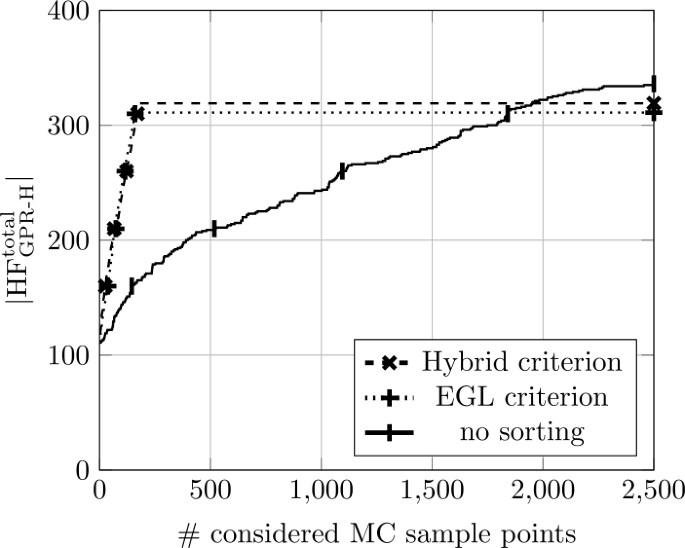Figure 3

In the following, we compare these results to the results of the stochastic collocation hybrid approach proposed in . The hybrid method is the same, the difference lies in the choice of the surrogate model and the error indicator for defining the critical sample points. In , the surrogate model is built using an adaptive stochastic collocation approach with Leja nodes, which led to a maximum polynomial degree of three. Once the polynomial surrogate is built, it is not straightforward to update it during the estimation procedure. Thus, higher accuracy in the initial model is required. An adjoint error indicator is used to estimate the error of the surrogate model. Analogously to the standard deviation of the GPR model, this error indicator, multiplied with a safety factor. Using this stochastic collocation hybrid approach, the same accuracy, i.e., the same yield estimator, was reached using $$|\text{HF}_{\text{SC-H}}^{\text{total}}| = |\text{HF}_{\text{SC-H}}^{ \text{offline}}| + |\text{HF}_{\text{SC-H}}^{\text{online}}| = 330 + 165 = 495$$ high fidelity evaluations. The number of training data points was chosen such that the method performs best.

### Comparison with a linearization approach

In practice, often a simple linearization of the QoI is used for the MC analysis, assuming that the design parameter deviations are small enough to obtain valid results . Therefore we compare the proposed GPR-Hybrid approach with linearization in the following. Linearizations means here, that we use a surrogate model, built by linear interpolation with two points in each dimension, i.e., in addition to $$\mathbf {p}^{0} = [p^{0}_{1},p^{0}_{2},p^{0}_{3},p^{0}_{4} ]^{ \top }$$ we consider the four nodes

$$\mathbf {p}^{k} = \mathbf {p}^{0} + \delta _{\mathbf {p}} \mathbf {e}_{k},\quad k=1,\dots ,4,$$

where $$\mathbf {e}_{k}$$ is the k-th unit vector and $$\delta _{\mathbf {p}}>0$$ the step size (if interpreted in the context of finite differences). Alternatively, derivative information could be used if available. These five nodes are used to create a linear approximation according to

$$\tilde{S}_{\text{GPR},\omega _{j}}\bigl(\mathbf {p}^{k}\bigr) = \sum _{l=1}^{| \mathbf {p}^{k}|} \bigl(a_{l} \mathbf {p}^{k}_{l} \bigr) + a_{| \mathbf {p}^{k}|+1},$$

where $$|\mathbf {p}^{k}|$$ is the length of the vector $$\mathbf {p}^{k}$$ and the $$a_{l}$$ are the coefficients of the linearization. This model is setup for each frequency point $$\omega _{j}$$ and for the real and the imaginary part of the S-parameter separately. Then a MC analysis on the linear surrogate models is performed. In Fig. 4 we see the results of the yield estimation for different values of $$\delta _{\mathbf {p}}$$. We compare this to the MC solution on the high fidelity model as reference solution and the GPR-Hybrid solution from Sect. 4.1. We introduce $$\upsilon \in [0,1]$$ as a measure of the magnitude of deviation. The covariance matrix Σ is multiplied with this factor υ in order to obtain problem settings with varying magnitude of uncertainty, i.e., we consider $$\mathbf {p}\sim {\mathcal{N_{T}} ( \overline{\mathbf {p}}, \upsilon \boldsymbol {\Sigma },\mathbf {lb} , \mathbf {ub} )}$$ with different values for υ. For $$\upsilon = 1$$ we obtain the results of Sect. 4.1, for $$\upsilon <1$$ the scaled variance decreases and the yield estimator increases until for $$\upsilon =0$$ there is no uncertainty at all and the yield is $$Y=1$$ since $$\overline{\mathbf {p}}$$ is in the safe domain. While the GPR-Hybrid solution exactly matches the reference solution for all magnitudes υ of uncertainty, we observe considerable deviations in the linearization model for any value of $$\delta _{\mathbf {p}}$$ (for $$\upsilon >0.5$$). These deviations decrease as expected with decreasing variance.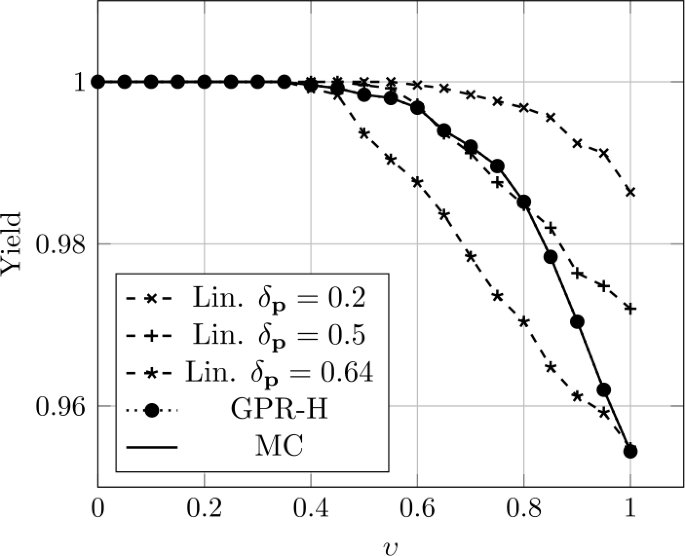Figure 4

### Lowpass filter

We consider as industrial example a stripline lowpass filter, see Fig. 5, taken from the examples library of CST Studio Suite®. We consider six uncertain geometrical parameters $$\mathbf {g} = [L_{1},L_{2},L_{3},W_{1},W_{2},W_{3}]^{\top }$$ describing length and width of the single blocks. Again, we assume the uncertain parameters to follow a truncated Gaussian distribution with mean and covariance (in mm) given by

$$\overline{\mathbf {g}} = [6.8, 5.1, 9.0, 1.4, 1.4, 1.3]^{\top } \quad \text{and}\quad \boldsymbol {\Sigma _{\mathbf {g}}} = \operatorname{diag} \bigl( \bigl[0.3^{2}, 0.3^{2}, 0.3^{2}, 0.1^{2}, 0.1^{2}, 0.1^{2}\bigr] \bigr).$$

The distribution of $$L_{1}$$, $$L_{2}$$ and $$L_{3}$$ is truncated at $$L_{i}\pm 3\text{ mm}$$ ($$i=1,2,3$$), the distribution of $$W_{1}$$, $$W_{2}$$ and $$W_{3}$$ at $$W_{i}\pm 0.3\text{ mm}$$ ($$i=1,2,3$$). Since the requirement for a low pass filter is to allow low frequency signals to pass through while filtering out high frequency signals, in this example we have two performance feature specifications given by

\begin{aligned} \text{I.) }&\quad \bigl\vert S_{\omega }(\mathbf {g}) \bigr\vert \stackrel{!}{\geq } -1 \text{ dB}\quad \forall \omega \in T_{\omega ,1} = [ 2 \pi 0, 2 \pi 4 ] \text{ in GHz}, \\ \text{II.) }&\quad \bigl\vert S_{\omega }(\mathbf {g}) \bigr\vert \stackrel{!}{\leq } -20 \text{ dB}\quad \forall \omega \in T_{\omega ,2} = [ 2 \pi 5, 2 \pi 7 ] \text{ in GHz}. \end{aligned}

As in the previous example we set $$\tilde{\sigma }_{Y}=0.01$$ which leads to a sample size of $$N_{\mathrm {MC}}= 2500$$, according to (8). Again, we show test results for $$N_{\text{B}}=50$$, $$N_{\text{B}}=20$$ and $$N_{\text{B}}=1$$ and $$\varepsilon _{t}=0$$. Also, the kernel function and the hyperparameter settings are as in the previous example. The safety is set to $$\gamma =3$$. Further we consider eight equidistant frequency points $$\omega _{j} \in T_{\text{d}}$$, i.e., eight GPR surrogate models are built. The evaluation of the high fidelity model is implemented in CST, using the default parameters of the frequency domain solver. The mathematical model is described in . An evaluation within CST calculates the S-parameter in a whole frequency range, i.e., for all considered frequency points $$\omega _{j} \in T_{\text{d}}$$. Therefore, with respect to this example, we look at the number of CST calls $$|\text{CC}_{ \text{method}}|$$ as a measure for the computational effort. As before, in order to measure the efficiency for parallel computing, we introduce the number of effective calls $$|\text{EC}_{\text{method}}| = \lceil \frac{|\text{CC}_{\text{method}}|}{N_{\text{B}}} \rceil$$ as the number of non-parallelizable CST calls. As reference value we consider the yield estimation with a pure Monte Carlo analysis. There, the computational effort is given by $$|\text{CC}_{\text{Ref.}}| = 2500$$ and the estimated yield is $$\tilde{Y}_{\text{Ref.}} = 87.08\%$$. Again, the size of the initial training data set in the GPR-Hybrid approach has been chosen such that the total costs are minimal. This leads to an initial training data set of $$|\mathcal{T}_{\text{I}}|=30$$ sample points. This means we have an offline cost of $$|\text{CC}_{\text{GPR-H}}^{ \text{offline}}|=30$$, because all frequency points are evaluated simultaneously in CST. Now we evaluate the $$N_{\mathrm {MC}}$$ sample points on the GPR model. If one sample point for one frequency point turns out to be a critical sample point, we evaluate this sample point for all frequency points with CST and use this information also for a possible update of the GPR models.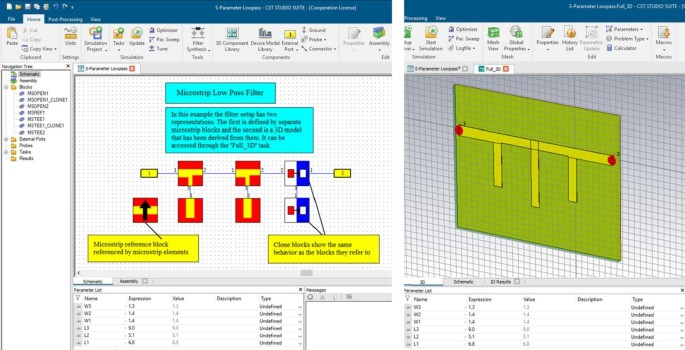Figure 5

Table 3 shows the online CST calls $$|\text{CC}_{\text{GPR-H}}^{\text{online}}|$$ and effective calls $$|\text{EC}_{\text{GPR-H}}^{\text{online}}|$$ for different updating settings. Compared to classic MC analysis the computational effort can be reduced by a factor between 6 and almost 9, depending on the settings, while maintaining the accuracy. The lower savings in computational effort compared to the previous example of the waveguide is due to the fact that it is a more complex example on the one hand, but on the other hand also due to the simultaneous evaluation of all frequency points, because often a sample point is not critical for all frequency points. The results regarding the impact of the batch size $$N_{\text{B}}$$ remains similar as in the previous example. Without using batches, the lowest number of CST calls is needed. The number increase with the size of the batch, while the costs for effective calls decrease using parallel computations. Also, a slight improvement of efficiency by sorting the sample points could be observed.

## Conclusions

A hybrid approach combining the efficiency of surrogate based approaches and the reliability and accuracy of the classic Monte Carlo method has been proposed. As surrogate model Gaussian Process Regression has been introduced and its standard deviation estimator was used as error indicator. Numerical results show that the computational effort can be significantly reduced while maintaining accuracy. This allows yield estimation in a reasonable time without the need for high performance computers as it would be the case with a pure Monte Carlo analysis. Future research will focus on embedding the presented yield estimation methods in yield optimization. Furthermore, interpolation in the direction of the range parameter could be investigated.

## Abbreviations

PDE:

partial differential equation

FEM:

finite element method

MC:

Monte Carlo

IS:

Importance Sampling

RBF:

radial basis functions

GPR:

Gaussian process regression

QoI:

quantity of interest

GP:

Gaussian process

## References

1. 1.

Graeb HE. Analog design centering and sizing. Dordrecht: Springer; 2007.

2. 2.

Hammersley JM, Handscomb DC. Monte Carlo methods. London: Methuen & Co Ltd; 1964.

3. 3.

Rao CR, Toutenburg H. Linear models: least squares and alternatives. 2nd ed. New York: Springer; 1999.

4. 4.

Rasmussen CE, Williams CKI. Gaussian processes for machine learning. Cambridge: MIT Press; 2006.

5. 5.

Babuška I, Nobile F, Tempone R. A stochastic collocation method for elliptic partial differential equations with random input data. SIAM J Numer Anal. 2007;45(3):1005–34. https://doi.org/10.1137/100786356.

6. 6.

Li J, Xiu D. Evaluation of failure probability via surrogate models. J Comput Phys. 2010;229(23):8966–80. https://doi.org/10.1016/j.jcp.2010.08.022.

7. 7.

Butler T, Wildey T. Utilizing adjoint-based error estimates for surrogate models to accurately predict probabilities of events. Int J Uncertain Quantificat. 2018;8(2):143–59. https://doi.org/10.1615/Int.J.UncertaintyQuantification.2018020911.

8. 8.

Fuhrländer M, Georg N, Römer U, Schöps S. Yield optimization based on adaptive Newton-Monte Carlo and polynomial surrogates. Int J Uncertain Quantificat. 2020;10(4):351–73. https://doi.org/10.1615/Int.J.UncertaintyQuantification.2020033344.

9. 9.

Bect J, Ginsbourger D, Li L, Picheny V, Vazquez E. Sequential design of computer experiments for the estimation of a probability of failure. Stat Comput. 2012;22(3):773–93.

10. 10.

Bect J, Li L, Vazquez E. Bayesian subset simulation. SIAM/ASA J Uncertain Quantificat. 2017;5(1):762–86. https://doi.org/10.1137/16m1078276.

11. 11.

Xiao S, Oladyshkin S, Nowak W. Reliability analysis with stratified importance sampling based on adaptive kriging. Reliab Eng Syst Saf. 2020;197:106852.

12. 12.

Zhang J, Taflanidis AA. Accelerating MCMC via kriging-based adaptive independent proposals and delayed rejection. Comput Methods Appl Mech Eng. 2019;355:1124–47.

13. 13.

Dassault Systèmes Deutschland GmbH: CST Studio Suite®. 2018. www.3ds.com.

14. 14.

Jackson JD. Classical electrodynamics. 3rd ed. New York: Wiley; 1998. https://doi.org/10.1017/CBO9780511760396.

15. 15.

Nédélec JC. Mixed finite elements in R3. Numer Math. 1980;35(3):315–41. https://doi.org/10.1007/BF01396415.

16. 16.

Monk P. Finite element methods for Maxwell’s equations. Oxford: Oxford University Press; 2003.

17. 17.

Pozar DM. Microwave engineering. New York: Wiley; 2011. https://books.google.at/books?id=_YEbGAXCcAMC.

18. 18.

Cohen AC. Truncated and censored samples: theory and applications. 2016. p. 1–303.

19. 19.

Wilhelm S, Manjunath B. tmvtnorm: a package for the truncated multivariate normal distribution. R J. 2010;2:25–9. https://doi.org/10.32614/RJ-2010-005.

20. 20.

Giles MB. Multilevel Monte Carlo methods. Acta Numer. 2015;24:259–328. https://doi.org/10.1017/S09624929.

21. 21.

Gustavsen B, Semlyen A. Rational approximation of frequency domain responses by vector fitting. IEEE Trans Power Deliv. 1999;14(3):1052–61. https://doi.org/10.1109/61.772353.

22. 22.

Kumar UD, Crocker J, Chitra T, Saranga H. Reliability and six sigma. EngineeringPro collection. New York: Springer; 2006. https://books.google.td/books?id=5_amcGFkhEIC.

23. 23.

Echard B, Gayton N, Lemaire M. AK-MCS: an active learning reliability method combining kriging and Monte Carlo simulation. Struct Saf. 2011;33(2):145–54.

24. 24.

Pedregosa F, Varoquaux G, Gramfort A, Michel V, Thirion B, Grisel O, Blondel M, Prettenhofer P, Weiss R, Dubourg V, Vanderplas J, Passos A, Cournapeau D, Brucher M, Perrot M, Duchesnay E. Scikit-learn: machine learning in Python. J Mach Learn Res. 2011;12:2825–30.

25. 25.

Loukrezis D. Benchmark models for uncertainty quantification. GitHub. 2019. https://github.com/dlouk/UQ_benchmark_models/tree/master/rectangular_waveguides/debye1.py.

26. 26.

Eller M, Reitzinger S, Schöps S, Zaglmayr S. A symmetric low-frequency stable broadband Maxwell formulation for industrial applications. SIAM J Sci Comput. 2017;39(4):703–31. https://doi.org/10.1137/16M1077817.

Download references

### Acknowledgements

The work of Mona Fuhrländer is supported by the Excellence Initiative of the German Federal and State Governments and the Graduate School of Computational Engineering at TU Darmstadt. The authors would like to thank Frank Mosler of Dassault Systèmes Deutschland GmbH for the fruitful discussions regarding the setup of the industrial example. Further, the authors thank Julien Bect of CentraleSupélec for very interesting discussions on generating and sorting training data for GPR models. Open Access funding enabled and organized by Projekt DEAL.

### Availability of data and materials

The codes and datasets generated and analyzed during the current study are available in the following GitHub repository https://github.com/temf/YieldEstOptGPR.

## Funding

No funding to report.

## Author information

Authors

### Contributions

All authors have jointly carried out research and worked together on the manuscript. The numerical tests have been conducted by MF. All authors read and approved the final manuscript.

### Corresponding author

Correspondence to Mona Fuhrländer.

## Ethics declarations

### Competing interests

The authors declare that they have no competing interests.

## Rights and permissions

Open Access This article is licensed under a Creative Commons Attribution 4.0 International License, which permits use, sharing, adaptation, distribution and reproduction in any medium or format, as long as you give appropriate credit to the original author(s) and the source, provide a link to the Creative Commons licence, and indicate if changes were made. The images or other third party material in this article are included in the article’s Creative Commons licence, unless indicated otherwise in a credit line to the material. If material is not included in the article’s Creative Commons licence and your intended use is not permitted by statutory regulation or exceeds the permitted use, you will need to obtain permission directly from the copyright holder. To view a copy of this licence, visit http://creativecommons.org/licenses/by/4.0/.

Reprints and Permissions